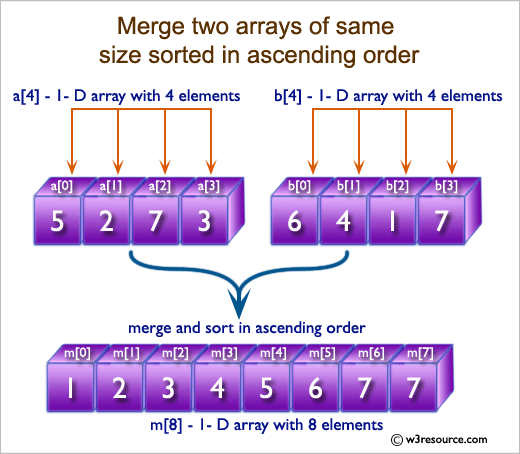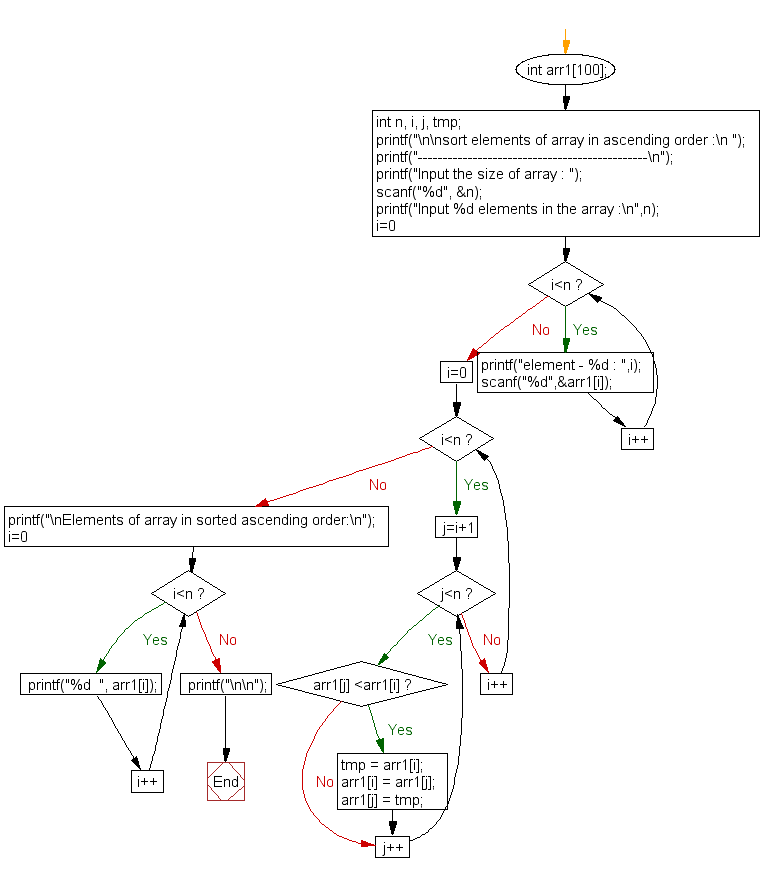﻿ C exercises: Sort elements of array in ascending order - w3resource# C Exercises: Sort elements of array in ascending order

## C Array: Exercise-11 with Solution

Write a program in C to sort elements of array in ascending order.

Pictorial Presentation:Sample Solution:

C Code:

``````#include <stdio.h>

void main()
{
int arr1;
int n, i, j, tmp;

printf("\n\nsort elements of array in ascending order :\n ");
printf("----------------------------------------------\n");

printf("Input the size of array : ");
scanf("%d", &n);

printf("Input %d elements in the array :\n",n);
for(i=0;i<n;i++)
{
printf("element - %d : ",i);
scanf("%d",&arr1[i]);
}

for(i=0; i<n; i++)
{
for(j=i+1; j<n; j++)
{
if(arr1[j] <arr1[i])
{
tmp = arr1[i];
arr1[i] = arr1[j];
arr1[j] = tmp;
}
}
}
printf("\nElements of array in sorted ascending order:\n");
for(i=0; i<n; i++)
{
printf("%d  ", arr1[i]);
}
printf("\n\n");
}
```
```

Sample Output:

```sort elements of array in ascending order :
----------------------------------------------
Input the size of array : 5
Input 5 elements in the array :
element - 0 : 2
element - 1 : 7
element - 2 : 4
element - 3 : 5
element - 4 : 9

Elements of array in sorted ascending order:
2  4  5  7  9
```

Flowchart:C Programming Code Editor:

Improve this sample solution and post your code through Disqus.

What is the difficulty level of this exercise?

﻿